# Pointer Arithmetic in C++

## Pointer Arithmetic in C++ with Examples:

In this article, I am going to discuss Pointer Arithmetic in C++ Language with examples. Please read our previous articles, where we discussed Dynamic Memory Allocation in C++ with examples. As we already discuss pointers are also a type of variable but instead of value, it stores address. So similar to operations performed on variables, pointers also support 4 arithmetic operations.

1. Pointer increment.
2. Pointer decrement.
4. Pointer subtraction by constant.
5. Difference between two pointers to calculate distance between pointer.
##### Pointer Arithmetic Examples in C++:

Let us understand with an example.

int A = {2, 4, 6, 8, 10};

int *p = A;

Here we have taken an example of an array of type ‘int’ of the size of five and with some elements. Also, we have taken a pointer ‘p’ and this is pointing on this array.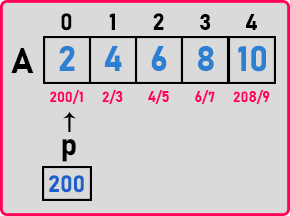We have assumed that the addresses are 200/1, 202/3, and so on. And the ‘p’ is a pointer which is having address 200 because I have assigned pointer ‘p’ to array ‘A’. This pointer is pointing to the beginning address of ‘A’. Now we can access this array by name ‘A’ as well as with the help of pointer ‘p’. Let us see what are the operations allowed using pointers, so there is a total of five operations allowed using pointers.

##### 1st operation: p++:

‘p++’ means, the pointer will move to the next location of the array. Here the address inside the pointer is 200. Then it will move to the next location.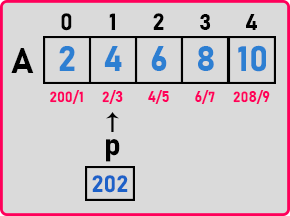Now, ‘p’ is pointing to the element of address ‘202’. We don’t mean adding one to the address, we mean moving the pointer to the next location. Just like when we say C++, it doesn’t mean that we want to add one to the C language. We want to move to the next step in C and that is object-oriented C language. By ‘++’ we can move the pointer forward.

It is an integer pointer so it has incremented by 2 bytes, when it was 200 then it became 202, if it is float pointer then it will move by 4 bytes because float takes 4 bytes and if it is a character pointer then it will move by 1 byte so, how many bytes pointer will move forward, it depends on the data type of that pointer.

##### 2nd operation: p–:

‘p–‘ means, the pointer will move backward. Now, ‘p’ is on ‘202’ and then ‘p–‘ will come on ‘200’.‘p–‘ is the same as ‘p++’. In ‘p–‘, the pointer will move backward and in ‘p++’, the pointer will move forward.

##### 3rd operation: p = p + c:

Suppose the pointer is pointing on 202 right now. Then if you say, p = p + 2; It will move by 2 integers or 2 elements in the array. So, where the pointer will go now? The pointer will be pointing on ‘206’.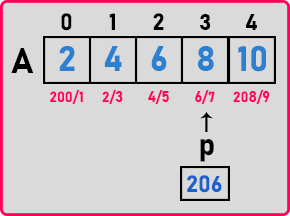So, this will move the pointer forward by 2 elements.

##### 4th operation: p = p – c:

p = p – 2, it moves the pointer backward by 2 elements. So here we can write any constant value at ‘c’.

##### 5th operation: d = q – p:

int *p = A;

int *q = &A;

We have a pointer ‘p’ that is pointing at ‘200’. Again, we have one more pointer that is ‘q’ that is pointing on 206. Here we have declared pointer ‘q’ that is pointing on & A.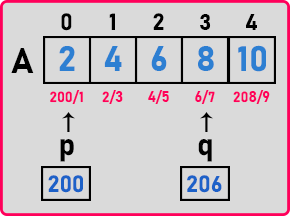So ‘q’ is pointing at 206. Now we can say q – p and take it in some variable as

d = q – p;

So, what is the result? What happens when you subtract two pointers q – p? That is 206 – 200. This will give 6 but it is divided by two. Why we are dividing it by 2? Because these are integers and their size is 2. So, the answer is 3.

d = q – p = 206 – 200 = 6

d = 6 /2 = 3

d = 3

So ‘d’ is 3.

So, we get to know the distance between two pointers. So, this gives the distance between two pointers or the number of elements in between two pointers. We have subtracted the addresses of two elements and divided the result by the size of the data type. Then that’s all we get the number of elements between two pointers.

Now if we say, d = p – q, then,

d = 200 – 206;

d = -6 / 2 = -3

d = -3

Here d is negative. So, this negative means that the ‘p’ pointer is nearer and the ‘q’ pointer is farther. If I’m getting a positive result then it means the first pointer is far away. If I’m getting a negative result, then it means the second pointer is farther. So that’s all only 5 arithmetic operations are allowed on the pointer.

Apart from this, we cannot perform any operation like the addition of 2 pointers or multiplication or division because that doesn’t make any sense. Like here ‘++’ was making sense that you go to the next element and ‘–’ will bring the pointer back to the previous element or move this element away in forward and backward then give the difference between two pointers. These are making sense so that’s the reason only these five operations are there.

##### Program on Operations on Pointers using C++:
```#include <iostream>
using namespace std;
int main()
{
int A = { 2, 4, 6, 8, 10 };
int *p = A;
int *q = &A;

cout << "Addresses of elements of the Array:\n";
for (int i = 0; i < 5; i++)
{
cout << A + i << endl;
}

cout << "\nOperations:\n";
p++;
cout << "p++:" << p << endl;
p--;
cout << "p--:" << p << endl;
p = p + 2;
cout << "p = p + 2:" << p << endl;
p = p - 2;
cout << "p = p - 2:" << p << endl;
int d = q - p;
d = d / 2;
cout << "d = q - p:" << d << endl;
}
```
###### Output: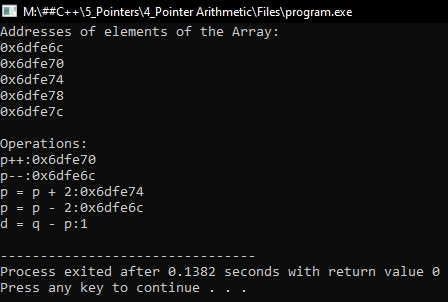Note: Pointer multiplication, division, and modulo are not allowed. I will explain all the operations by taking one example:

Let’s take an array

int array={1,2,3,4,5}

int *ptr=array; //ptr is pointing to the first element of an array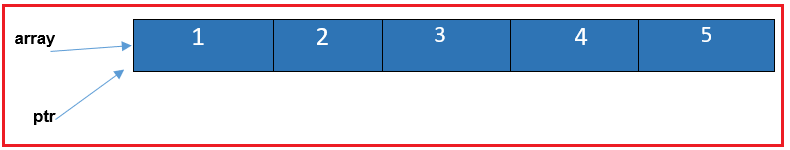##### Pointer Increment in C++:

Now let’s perform pointer increment:

int array={1,2,3,4,5}

int *ptr=array;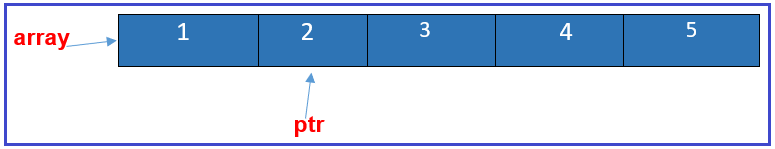##### Pointer Decrement in C++:

int array={1,2,3,4,5}
int *ptr=array;
ptr++;
ptr–;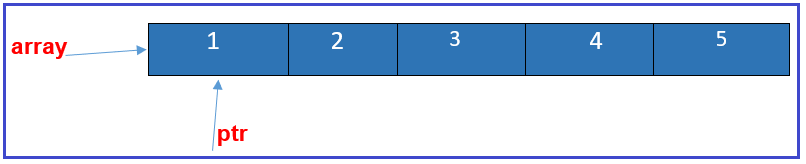Note: pointer decrement wont decrement value 1 from address instead it moves to the previous index

int array={1,2,3,4,5}
int *ptr=array;
ptr++;
ptr–;
ptr=ptr+3; //moves the index by 3 and hence now it is pointing to value 4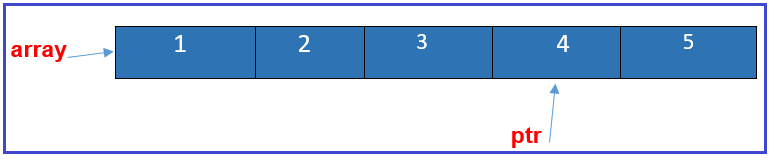##### Pointer Subtraction by Constant:

int array={1,2,3,4,5}
int *ptr=array;
ptr++;
ptr–;
ptr=ptr+3;
ptr=ptr-2; //Decrement the index position by 2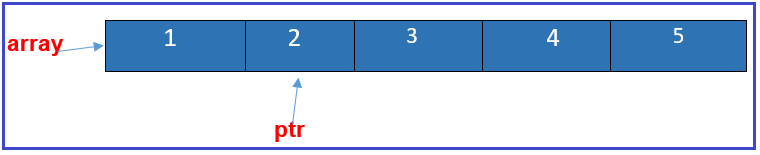##### Pointer Distance Between Two Pointers in C++

Let’s say I have created another pointer which is pointing to the first element of the array to calculate the distance between two pointers we perform the following operation.
int array={1,2,3,4,5}
int *ptr=array;
ptr++;
ptr–;
ptr=ptr+3;
ptr=ptr-2
int *ptr1=array;
int distance=ptr-ptr1;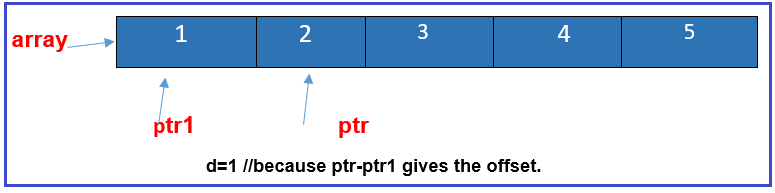1.